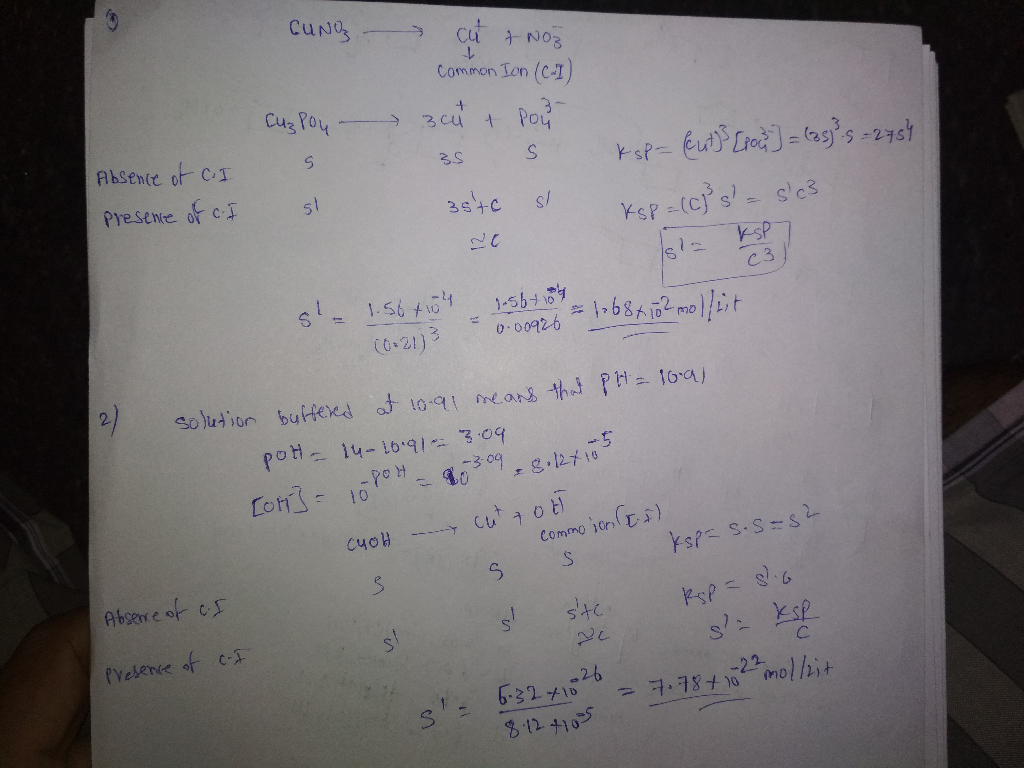# What is the molar solubility (in mol/L) of Cu3PO4 (Ksp = 1.56⋅10−4) in a 0.21 M CuNO3 solution. W...

What is the molar solubility (in mol/L) of Cu3PO4 (Ksp = 1.56⋅10−4) in a 0.21 M CuNO3 solution.

What is the molar solubility (in mol/L) of CuOH (Ksp = 6.32⋅10−26) in a solution buffered at 10.91.##### Add Answer of: What is the molar solubility (in mol/L) of Cu3PO4 (Ksp = 1.56⋅10−4) in a 0.21 M CuNO3 solution. W...
More Homework Help Questions Additional questions in this topic.

• #### Calculate the molar solubility of CaF2 in a solution containing 0.357 M of Ca(NO3)2. The Ksp valu...

Need Online Homework Help?